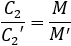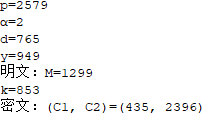GitHub

# ELGamal密码

ELGamal密码是除了RSA之外最有代表性的公开密钥密码之一，它的安全性建立在离散对数问题的困难性之上，是一种公认安全的公钥密码。

## 离散对数问题

设p为素数，若存在一个正整数α，使得α、α2、...、αp-1关于模p互不同余，则称α为模p的一个原根。于是有如下运算：

α的幂乘运算：

y=αx(mod p)，1≤x≤p-1

α的对数运算：

x=logαy，1≤y≤p-1

只要p足够大，求解离散对数问题时相当复杂的。离散对数问题具有较好的单向性。

# ELGamal加解密算法

1.随机地选择一个大素数p，且要求p-1有大素数因子，将p公开。

2.选择一个模p的原根α，并将α公开。

3.随机地选择一个整数d（1＜d＜p-1）作为私钥，并对d保密。

4.计算公钥y=αd(mod p)，并将y公开。

## 加密

1.随机地选取一个整数k（1＜k＜p-1）。

2.计算U=yk(mod p)、C1k(mod p)、C2=UM(mod p)。

3.取(C1,C2)作为密文。

## 解密

1.计算V=C1d(mod p)。

2.计算M=C2V-1(mod p)。

# ELGamal算法细节

实现ELGamal算法，需要实现以下几个部分：

1.对大数的素数判定；

2.判断原根；

3.模指运算；

4.模逆运算。

## 判断原根

已知a和m互素，如果d是满足ad=1(mod m)的最小正整数，则称d为a模m的阶，记为d=σm(a)。由于a和m互素，根据欧拉定理可知aφ(m)=1(mod m)，由此可以得到σm(a) | φ(m)。

若a是m的原根，则σm(a)=φ(m)。

根据上述两点，推出逆否命题：如果∃d | φ(m)且d≠φ(m)，使得ad=1(mod m)，则a不是模m的原根。所以判断a是否为模m的原根，最快的方法就是判断φ(m)的每一个因子d是否使得ad=1(mod m)。如果满足ad=1(mod m)的d=φ(m)，则a是模m的原根。

e.m.判断2是不是模11的原根

φ(11)=10

10的因子有1、2、5、10，所以：

2(mod 11)=2

22(mod 11)=4

25(mod 11)=10

210(mod 11)=1

因此，2是模11的原根。

# ELGamal密码的安全性

由于ELGamal密码的安全性建立在GF(p)上离散对数的困难性之上，而目前尚无求解GF(p)上离散对数的有效算法，所以在p足够大时ELGamal密码是安全的。理想情况下p为强素数，p-1=2q，q为大素数。

为了安全加密所使用的k必须是一次性的。如果长期使用同一个k加密的话，就可能被攻击者获取，从而根据V=U=yk(mod p)，M=C2V-1(mod p)而得到明文。另外，使用同一个k加密不同的明文M和M'，则由于# Java实现

## ELGamal

 1 do {
2     p = BigInteger.probablePrime(100, new Random());
3 } while (p.subtract(BigInteger.ONE).divide(new BigInteger("2")).isProbablePrime(100));
4 do {
5     alpha = new BigInteger(100, new Random());
6 } while (! isOrigin(alpha, p));
7 do {
8     d = new BigInteger(100, new Random());
9 } while (d.compareTo(BigInteger.ONE) != 1 || d.compareTo(p.subtract(BigInteger.ONE)) != -1);
10 y = alpha.modPow(d, p);
 1 /**
2  * 加密
3  * @param M
4  * @return
5  */
6 BigInteger[] encrypt(BigInteger M) {
7     BigInteger[] C = new BigInteger;
8     BigInteger k, U;
9     do {
10         do {
11             k = new BigInteger(100, new Random());
12         } while (k.compareTo(BigInteger.ONE) != 1 || k.compareTo(p.subtract(BigInteger.ONE)) != -1);
13         U = y.modPow(k, p);
14     } while (U.intValue() != 1);
15     C = alpha.modPow(k, p);
16     C = U.multiply(M).mod(p);
17     return C;
18 }
 1 /**
2  * 解密
3  * @param C
4  * @return
5  */
6 BigInteger decrypt(BigInteger[] C) {
7     BigInteger V = C.modPow(d, p);
8     BigInteger M = C.multiply(V.modPow(new BigInteger("-1"), p)).mod(p);
9     return M;
10 }

## 判断原根

 1 /**
2  * 判断a是否为模m的原根，其中m为素数
3  * @param a
4  * @param m
5  * @return
6  */
7 static boolean isOrigin(BigInteger a, BigInteger m) {
8     if (a.gcd(m).intValue() != 1) return false;
9     BigInteger i = new BigInteger("2");
10     while (i.compareTo(m.subtract(BigInteger.ONE)) == -1) {
11         if (m.mod(i).intValue() == 0) {
12             if (a.modPow(i, m).intValue() == 1)
13                 return false;
14             while (m.mod(i).intValue() == 0)
15                 m = m.divide(i);
16         }
17         i = i.add(BigInteger.ONE);
18     }
19     return true;
20 }

# 测试

p=2579

α=2

d=765

M=1299

k=853

## 测试结果# 参考文献

张焕国，唐明.密码学引论（第三版）.武汉大学出版社，2015年

posted @ 2019-01-24 17:28  蒙丿鑫  阅读(3526)  评论(0编辑  收藏